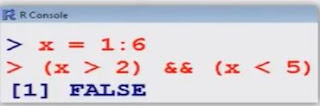# Basic calculations: Logical operators in R Languages

Logical Operators and Comparisons

The following table shows the operations and functions for logical comparisons (True or False).

Examples :

> x = 1 : 6       # Generates x=1,2,3,4,5,6
> (x > 2) & (x < 5)  # Checks whether the values are greater than 2 and less than 5

  FALSE  FALSE  TRUE  TRUE  FALSE  FALSE

> x [(x > 2) & (x < 5)]  # Finds which values are greater than 2 and smaller than 5.
   3  4

Logical Operators and Comparisons

• The shoter form performs element-wise comparisons in almost the same way as arithmetic operators.
• The longer from evaluates left to right examining only the first element of each vector. Evaluation proceeds only until the result is determined.
Example of   " The longer form evaluates left to right examining only the first element of each vector"
> x = 1 : 6           # Generates x = 1,2,3,4,5,6> (x > 2)  && (x < 5)
  FALSE

is equivalent to:

> (x > 2) & (x < 5)
  FALSENote that x is only the first element in x.

> x[ (x > 2) && (x < 5) ]
integer(0)                # Finds which values are greater than 2 and smaller than 5
This statement is equivalent to

> x [ (x > 2) & (x < 5) ]
integer (0)